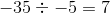Example Questions

Example Question #1 : Operations

Andrew spends every Saturday at the gym working out. He can complete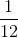of his workout inof an hour. If he continues at this rate, how much of his workout does Andrew complete per hour?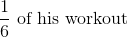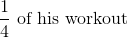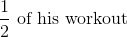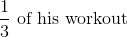Explanation:

The phrase "per hour" gives us a clue that we are going to divide. In this problem, we can replace the word "per" with a division sign; therefore, we will have the portion of his workout,, divided by hours,: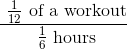Remember that when we divide fractions, we can simply multiply by the reciprocal of the denominator to solve.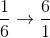Therefore: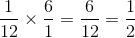Andrew can completeof his workout per hour.

Example Question #1 : Operations

Solve: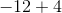Explanation:

In order to solve this problem, we need to start aton the number line.Next, we have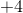which means we need to moveplaces to the right on the number line. When we have an addition sign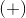we move to the right because that is towards the positive side of the number line. When we have a subtraction sign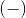we move to the left because that is towards the negative side of the number line.The orange arrow movedplaces to the right, and ended at; thus,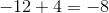Example Question #1 : Operations

Solve: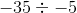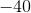Explanation:

We know the following information: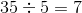In this particular case, do the negative numbers change our answer? . There are a couple of rules that we need to remember when multiplying with negative numbers:

• A negative number divided by a positive number will always equal a negative number, and a positive number divided by a negative number will always equal a negative number.
• A negative number divided by a negative number will always equal a positive number

Thus,